• 双线性插值算法的C语言实现方式，双线性插值算法的C语言实现方式 可以参考一下。
• 双线性插值法原理： ① 何为线性插值？ 插值就是在两个数之间插入一个数，线性插值原理图如下： 在位置 x 进行线性插值，插入的值为f(x) ↑ ② 各种插值法： 插值法的第一步都是相同的，计算目标图...
码字不易，如果此文对你有所帮助，请帮忙点赞、评论，感谢！

一. 双线性插值法原理：

① 何为线性插值？

插值就是在两个数之间插入一个数，线性插值原理图如下：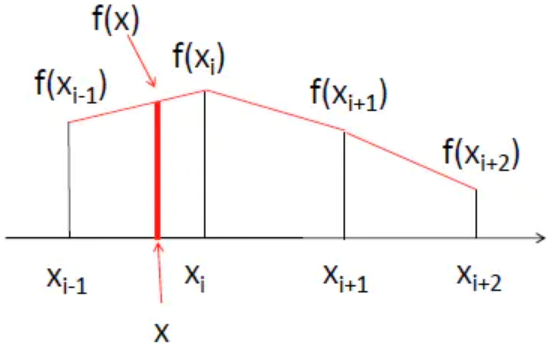在位置 x 进行线性插值，插入的值为f(x) ↑

② 各种插值法：

插值法的第一步都是相同的，计算目标图（dstImage）的坐标点对应原图（srcImage）中哪个坐标点来填充，计算公式为：

srcX = dstX * (srcWidth/dstWidth)

srcY = dstY * (srcHeight/dstHeight)

(dstX,dstY)表示目标图像的某个坐标点，(srcX,srcY)表示与之对应的原图像的坐标点。srcWidth/dstWidth 和 srcHeight/dstHeight 分别表示宽和高的放缩比。

那么问题来了，通过这个公式算出来的 srcX, scrY 有可能是小数，但是原图像坐标点是不存在小数的，都是整数，得想办法把它转换成整数才行。

不同插值法的区别就体现在 srcX, scrY 是小数时，怎么将其变成整数去取原图像中的像素值。

最近邻插值（Nearest-neighborInterpolation）：看名字就很直白，四舍五入选取最接近的整数。这样的做法会导致像素变化不连续，在目标图像中产生锯齿边缘。

双线性插值（Bilinear Interpolation）：双线性就是利用与坐标轴平行的两条直线去把小数坐标分解到相邻的四个整数坐标点。权重与距离成反比。

双三次插值（Bicubic Interpolation）：与双线性插值类似，只不过用了相邻的16个点。但是需要注意的是，前面两种方法能保证两个方向的坐标权重和为1，但是双三次插值不能保证这点，所以可能出现像素值越界的情况，需要截断。

③ 双线性插值算法原理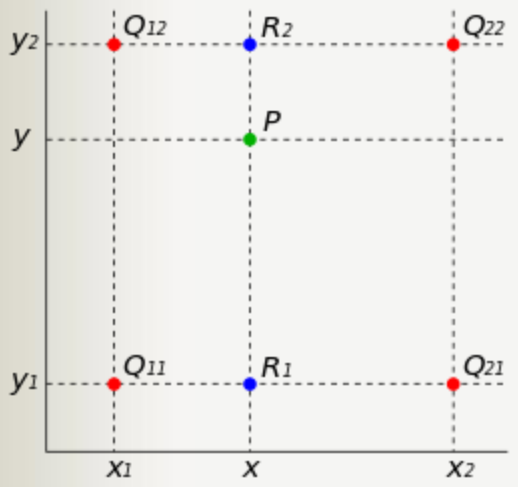双线性插值算法原理图，将P的小数坐标变成 Q11、Q12、Q21、Q22 4个整数坐标内容的加权和 ↑

假如我们想得到未知函数 f 在点 P = (x, y) 的值，假设我们已知函数 f 在 Q11 = (x1, y1)、Q12 = (x1, y2), Q21 = (x2, y1) 以及 Q22 = (x2, y2) 四个点的值。最常见的情况，f就是一个像素点的像素值。首先在 x 方向进行线性插值，然后再在 y 方向上进行线性插值，最终得到双线性插值的结果。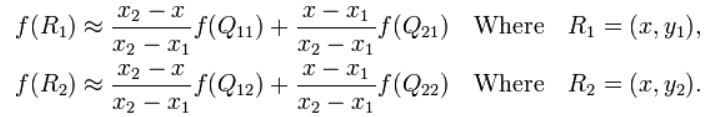x 方向上线性插值算法 ↑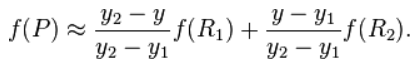y 方向上线性插值算法 ↑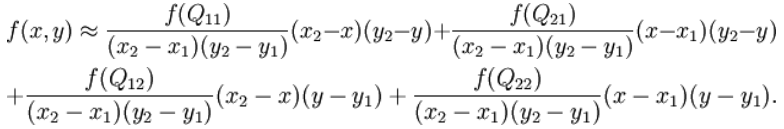双线性插值算法 ↑

④ 举例说明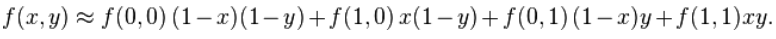如果选择一个坐标系统使得 f 的四个已知点坐标分别为 (0, 0)、(0, 1)、(1, 0) 和 (1, 1)，那么双线性插值公式就可以化简为上式 ↑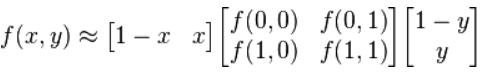矩阵表示为 ↑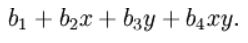可以看到，与这种插值方法名称不同的是，这种插值方法的结果通常不是线性的，它的形式是  ↑

二. python实现灰度图像双线性插值算法：

灰度图像双线性插值放大缩小

import numpy as np
import math
import cv2

def double_linear(input_signal, zoom_multiples):
'''
双线性插值
:param input_signal: 输入图像
:param zoom_multiples: 放大倍数
:return: 双线性插值后的图像
'''
input_signal_cp = np.copy(input_signal)   # 输入图像的副本

input_row, input_col = input_signal_cp.shape # 输入图像的尺寸（行、列）

# 输出图像的尺寸
output_row = int(input_row * zoom_multiples)
output_col = int(input_col * zoom_multiples)

output_signal = np.zeros((output_row, output_col)) # 输出图片

for i in range(output_row):
for j in range(output_col):
# 输出图片中坐标 （i，j）对应至输入图片中的最近的四个点点（x1，y1）（x2, y2），（x3， y3），(x4，y4)的均值
temp_x = i / output_row * input_row
temp_y = j / output_col * input_col

x1 = int(temp_x)
y1 = int(temp_y)

x2 = x1
y2 = y1 + 1

x3 = x1 + 1
y3 = y1

x4 = x1 + 1
y4 = y1 + 1

u = temp_x - x1
v = temp_y - y1

# 防止越界
if x4 >= input_row:
x4 = input_row - 1
x2 = x4
x1 = x4 - 1
x3 = x4 - 1
if y4 >= input_col:
y4 = input_col - 1
y3 = y4
y1 = y4 - 1
y2 = y4 - 1

# 插值
output_signal[i, j] = (1-u)*(1-v)*int(input_signal_cp[x1, y1]) + (1-u)*v*int(input_signal_cp[x2, y2]) + u*(1-v)*int(input_signal_cp[x3, y3]) + u*v*int(input_signal_cp[x4, y4])
return output_signal

# Read image
img = cv2.imread("../paojie_g.jpg",0).astype(np.float)
out = double_linear(img,2).astype(np.uint8)
# Save result
cv2.imshow("result", out)
cv2.imwrite("out.jpg", out)
cv2.waitKey(0)
cv2.destroyAllWindows()

三. 灰度图像双线性插值实验结果：原图 ↑放大2倍后图像 ↑

四. 彩色图像双线性插值python实现

from PIL import Image
import matplotlib.pyplot as plt
import numpy as np
import math
def BiLinear_interpolation(img,dstH,dstW):
scrH,scrW,_=img.shape
img=np.pad(img,((0,1),(0,1),(0,0)),'constant')
retimg=np.zeros((dstH,dstW,3),dtype=np.uint8)
for i in range(dstH):
for j in range(dstW):
scrx=(i+1)*(scrH/dstH)-1
scry=(j+1)*(scrW/dstW)-1
x=math.floor(scrx)
y=math.floor(scry)
u=scrx-x
v=scry-y
retimg[i,j]=(1-u)*(1-v)*img[x,y]+u*(1-v)*img[x+1,y]+(1-u)*v*img[x,y+1]+u*v*img[x+1,y+1]
return retimg
im_path='../paojie.jpg'
image=np.array(Image.open(im_path))
image2=BiLinear_interpolation(image,image.shape*2,image.shape*2)
image2=Image.fromarray(image2.astype('uint8')).convert('RGB')
image2.save('out.png')

五. 彩色图像双线性插值实验结果：原图 ↑RGB图像双线性插值放大2倍后图像 ↑

六. 最近邻插值算法和双三次插值算法可参考：

① 最近邻插值算法：https://www.cnblogs.com/wojianxin/p/12515061.html

https://blog.csdn.net/Ibelievesunshine/article/details/104936006

② 双三次插值算法：https://www.cnblogs.com/wojianxin/p/12516762.html

https://blog.csdn.net/Ibelievesunshine/article/details/104942406

七. 参考内容：

https://www.cnblogs.com/wojianxin/p/12516029.html

https://www.jianshu.com/p/84c7d98be5ee

展开全文数字图像处理 opencv
• 图像处理算法中的双线性插值算法的纯c++实现， 希望能给大家提供一些思路。
• JAVA双线性插值算法实现图片的缩写放大功能。本程序根据这个算法实现了图片的缩小功能，而且附带测试图片可以运行测试。
• 首先运用双线性插值算法，对低分辨率传感器所成图像进行升采样以提高图像分辨率，然后利用基于OpenCV标定算法对其进行标定，最后将标定结果与传统方法标定结果进行分析比较。实验结果表明，该方法能够将低分辨率...
• C#的二维数组双线性插值算法。 用于二维数组的双线性插值算法，可分别设置长度和宽度。
• 其中有两种简单又常用的插值算法用来实现图像缩放，分别是最近邻插值算法和双线性插值算法。 最近邻插值算法: 最近邻插值算法的思想十分简单 设原始图像src的高为h，宽为w，图像上像素值为(x,y)。 设目标图像dst...
图像缩放：

resize函数是在OpenCV中经常使用的函数，功能是将一副加载到Mat中的图像调整尺寸或者按照比例进行缩放。

其中有两种简单又常用的插值算法用来实现图像缩放，分别是最近邻插值算法和双线性插值算法。

最近邻插值算法:

最近邻插值算法的思想十分简单

设原始图像src的高为h，宽为w，图像上像素值为(x,y)。

设目标图像dst的高H，宽为W，图像上的像素值为(X,Y)。

那么根据比例缩放的思想有xX=wW$\frac{x}{X}=\frac{w}{W}$$\frac{x}{X}=\frac{w}{W}$
同理，纵向上的像素对应比值为yY=hH$\frac{y}{Y}=\frac{h}{H}$$\frac{y}{Y}=\frac{h}{H}$

那么在考虑目标图像dst上面的像素点(X,Y)对应到原始图像src上面像素点的位置为

(x,y)=(wWX,hHY)$\left(x,y\right)=\left(\frac{w}{W}X,\frac{h}{H}Y\right)$$(x,y)=(\frac{w}{W}X,\frac{h}{H}Y)$

这里要对wWX$\frac{w}{W}X$$\frac{w}{W}X$与hHY$\frac{h}{H}Y$$\frac{h}{H}Y$两个数值进行取整，在OpenCV源码当中，是用cvFloor函数取整，即舍去小数部分。

代码：

void InterNearestResize(const Mat &src, Mat &dst, Size &dsize, double fx = 0.0, double fy = 0.0)//原始图形以及缩放比例
{

Size ssize = src.size();//获取矩阵大小
CV_Assert(ssize.area() > 0);//保证矩阵的长宽都大于0

if (!dsize.area())//如果dsize为(0,0)
{
dsize = Size(saturate_cast<int>(src.cols*fx),//satureate_cast防止数据溢出
saturate_cast<int>(src.rows*fy));

CV_Assert(dsize.area());
}
else
{
fx = (double)dsize.width / src.cols;//Size中的宽高和mat中的行列是相反的
fy = (double)dsize.height / src.rows;
}

dst.create(Size(src.cols*fy,src.rows*fx), src.type());//将dst矩阵大小变为想要的尺寸

fx = 1.0 / fx;
fy = 1.0 / fy;
uchar *ps = src.data;
uchar *pd = dst.data;
int channels = src.channels(),x,y,out,in;

for (int row = 0; row < dst.rows; row++)
{
x = cvFloor(row * fx);
for (int col = 0; col < dst.cols; col++)
{
y = cvFloor(col * fy);
for (int c = 0; c < channels; c++)
{
out = (row * dst.cols + col) * channels + c;
in = (x * src.cols + y) * channels + c;
pd[out] = ps[in];
}

//dst.at<Vec3b>(row, col) = src.at<Vec3b>(x, y);
}
}
}

双线性插值算法:

如果原始图像src的大小是3×3，目标图像dst的大小是4×4，考虑dst中(1,1)点像素对应原始图像像素点的位置为(0.75,0.75)，如果使用最近邻算法来计算，原始图像的位置在浮点数取整后为坐标(0,0)。

上面这样粗暴的计算会丢失很多信息，考虑(0.75,0.75)这个信息，它表示在原始图像中的坐标位置，相比较取(0,0)点，(0.75,0.75)貌似更接近(1,1)点，那如果将最近邻算法中的取整方式改为cvRound（四舍五入）的方式取(1,1)点，同样会有丢的信息，即丢失了“0.25”部分的(0,0)点、(1,0)点和(0,1)点。

可以看到，dst图像上(X,Y)对应到src图像上的点，最好是根据计算出的浮点数坐标，按照百分比各取四周的像素点的部分。
如下图：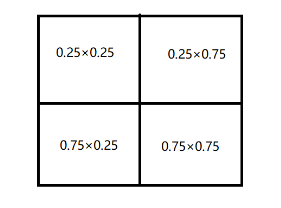双线性插值的原理相类似，这里不写双线性插值计算点坐标的方法，容易把思路带跑偏，直接就按照比率权重的思想考虑。

将(wWX,hHY)$\left(\frac{w}{W}X,\frac{h}{H}Y\right)$$(\frac{w}{W}X,\frac{h}{H}Y)$写成(x′+u,y′+v)$\left({x}^{\prime }+u,{y}^{\prime }+v\right)$$(x'+u,y'+v)$的形式，表示将x$x$$x$与y$y$$y$中的整数和小数分开表示uv$uv$$uv$分别代表小数部分。

这样，根据权重比率的思想得到计算公式

(X,Y)=(1−u)⋅(1−v)⋅(x,y)+(u−1)⋅v⋅(x,y+1)+u⋅(v−1)⋅(x+1,y)+(u⋅v)⋅(x,y)$\left(X,Y\right)=\left(1-u\right)·\left(1-v\right)·\left(x,y\right)+\left(u-1\right)·v·\left(x,y+1\right)+u·\left(v-1\right)·\left(x+1,y\right)+\left(u·v\right)·\left(x,y\right)$$(X,Y)=(1-u)·(1-v)·(x,y)+(u-1)·v·(x,y+1)+u·(v-1)·(x+1,y)+(u·v)·(x,y)$

在实际的代码编写中，会有两个问题，一个是图像会发生偏移，另一个是效率问题。

几何中心对齐：

由于计算的图像是离散坐标系，如果使用(wWX,hHY)$\left(\frac{w}{W}X,\frac{h}{H}Y\right)$$(\frac{w}{W}X,\frac{h}{H}Y)$公式来计算，得到的(X,Y)值是错误的比率计算而来的，即(x+1,y)、(x,y+1)、(x+1,y+1)这三组点中，有可能有几个没有参与到比率运算当中，或者这个插值的比率直接是错误的。
例如，src图像大小是a×a$a×a$$a×a$，dst图像的大小是0.5a×0.5a$0.5a×0.5a$$0.5a×0.5a$。

根据原始公式计算

(wW,hH)$\left(\frac{w}{W},\frac{h}{H}\right)$$(\frac{w}{W},\frac{h}{H})$得到(2,2)$\left(2,2\right)$$(2,2)$（注意这不是表示点坐标，而是x和y对应的比率）

如果要计算dst点(0,0)对应的插值结果，由于(2,2)$\left(2,2\right)$$(2,2)$是整数，没有小数，所以最后得到dst点在(0,0)$\left(0,0\right)$$(0,0)$点的像素值就是src图像上在(0,0)$\left(0,0\right)$$(0,0)$点的值。然而，我们想要的dst在(0,0)$\left(0,0\right)$$(0,0)$上的结果是应该是有(0,0)(1,0)(0,1)(1,1)这四个点各自按照0.5×0.5的比率加权的结果。
所以我们要将dst上面的点，按照比率(wW,hH)$\left(\frac{w}{W},\frac{h}{H}\right)$$(\frac{w}{W},\frac{h}{H})$向右下方向平移0.5个单位。
公式如下：
(x,y)=(XwW+0.5(wW−1),YhH+0.5(hH−1))$\left(x,y\right)=\left(X\frac{w}{W}+0.5\left(\frac{w}{W}-1\right),Y\frac{h}{H}+0.5\left(\frac{h}{H}-1\right)\right)$
(x,y)=(X\frac{w}{W}+0.5(\frac{w}{W}-1),Y\frac{h}{H}+0.5(\frac{h}{H}-1))

运算优化：

由计算公式可以得知，在计算每一个dst图像中的像素值时会涉及到大量的浮点数运算，性能不佳。

可以考虑将浮点数变换成一个整数，即扩大一定的倍数，运算得到的结果再除以这个倍数。

举一个简单的例子，计算0.25×0.75，可以将0.25和0.75都乘上8，得到2×6=12，结果再除以82${8}^{2}$$8^2$，这样运算的结果与直接计算浮点数没有差别。

在程序中，没有办法取得一个标准的整数，使得两个相互运算的浮点数都变成类似“2”和”6“一样的标准整数，只能取一个适当的值来尽量的减少误差，在源码当中取值为211${2}^{11}$$2^{11}$=2048，即2的固定幂数，最后结果可以通过用位移来表示除以一个2整次幂数，计算速度会有很大的提高。

代码：

void Inter_Linear(const Mat &src, Mat &dst, Size &dsize ,double fx = 0.0, double fy = 0.0)//双线性插值
{
Size ssize = src.size();//获取矩阵大小
CV_Assert(ssize.area() > 0);//保证矩阵的长宽都大于0

if (!dsize.area())//如果dsize为(0,0)
{
dsize = Size(saturate_cast<int>(src.cols*fx),//satureate_cast防止数据溢出
saturate_cast<int>(src.rows*fy));

CV_Assert(dsize.area());
}
else
{
fx = (double)dsize.width / src.cols;//Size中的宽高和mat中的行列是相反的
fy = (double)dsize.height / src.rows;
}

dst.create(dsize, src.type());

double ifx = 1. / fx;
double ify = 1. / fy;

uchar* dp = dst.data;
uchar* sp = src.data;

int iWidthSrc = src.cols;//宽(列数）
int iHiehgtSrc = src.rows;//高(行数)
int channels = src.channels();
short cbufy;
short cbufx;

for (int row = 0; row < dst.rows; row++)
{
float fy = (float)((row + 0.5) * ify - 0.5);
int sy = cvFloor(fy);//整数部分
fy -= sy;//小数部分
sy = std::min(sy, iHiehgtSrc - 2);
sy = std::max(0, sy);

cbufy = cv::saturate_cast<short>((1.f - fy) * 2048);
cbufy = 2048 - cbufy;

for (int col = 0; col < dst.cols; col++)
{
float fx = (float)((col + 0.5) * ifx - 0.5);
int sx = cvFloor(fx);
fx -= sx;

if (sx < 0)
{
fx = 0, sx = 0;
}
if (sx >= iWidthSrc - 1)
{
fx = 0, sx = iWidthSrc - 2;
}

cbufx = cv::saturate_cast<short>((1.f - fx) * 2048);
cbufx = 2048 - cbufx;

for (int k = 0; k < src.channels(); ++k)
{
dp[(row * dst.cols + col) * channels + k] =
(
sp[ ( sy * src.cols + sx ) * channels + k] * cbufx * cbufy +
sp[((sy + 1) * src.cols + sx) * channels + k] * cbufx * cbufy +
sp[(sy * src.cols + (sx + 1)) * channels + k] * cbufx * cbufy +
sp[((sy + 1) * src.cols + (sx + 1)) * channels + k] * cbufx * cbufy
) >> 22;
}
}
}

}

参考博客

对应OpenCV源码部分为
opencv-2.4.9\modules\imgproc\src\imgwarp 部分
展开全文图像缩放
• 九江332000)2(闽发证券有限责任公司上海200031)3(华为技术有限公司上海200121)摘要本文介绍了双线性插值算法在图像放缩领域的应用和具体实现的方法,并通过与传统放缩方法的比较,证明了该算法能显著提高图像缩放后的...
九江332000)2(闽发证券有限责任公司上海200031)3(华为技术有限公司上海200121)摘要本文介绍了双线性插值算法在图像放缩领域的应用和具体实现的方法,并通过与传统放缩方法的比较,证明了该算法能显著提高图像缩放后的清晰度。关键词图像处理双线性插值象素移交象素填充DOUBLELINEARINSERTINGALGORITHMBASEDIMAGEZOOMINGANDITSREALIZATIONWITHVC++FengHuijun1TaoSujuan2LiRong31(DepartmentofMathmatics&ComputerScience,JiujiangTeacherpsCollege,Jiujiang332000)2(MFSecuritiesCo.,Ltd.Shanghai200031)3(HuaweiTechnologiesCo.,Ltd.Shanghai200121)AbstractThisarticleintroducesaDoubleLinearInsertingAlgorithmbasedImageZooming(DLIAIZ)technique.TherealizationofDLIAIZwithVC++isproposed.ThecomparisonbetweentraditionalimagezoomingalgorithmandDLIAIZshowsthatthelatercangreatlyimprovethearticulationofzoomedimage.KeywordsImageprocessingDoublelinearinsertingPixelcarry-overPixelfilling1引言图像处理因其应用的广泛性,正受到越来越多的重视。在很多场合下,需要对图像进行缩放以改善视觉效果。使用VC++的MFC类库中的CDCJStretchBlt函数可以将位图进行放大或缩小,但在实际使用过程中发现用该函数进行放大会有很明显的失真现象。本文将基于BMP文件提出一种双线性插值算法,用以改进图像的放缩质量。最后给出了VC++中基于双线性插值算法的图像放缩技术的具体实现。2图像文件格式简介BMP图像文件格式有以下几个特点:Ó与VGA显示卡的高分辨率模式12h相对应,每个位平面由4位象素值表示,即它可以描述单色,16色,256色以及24位真彩色四种形式的图像信息。Ó与大部分图像文件格式不同,图像的扫描线是从下到上而不是从上到下的形式。ÓBMP文件支持行程编码压缩算法,但仅限于4位和8位象素值两种模式。ÓBMP文件的扫描线在每行末尾进行了规格化,使得BMP文件长度均能够被4整除。BMP文件由四部分组成:文件头、图像控制信息、调色板信息和位图点阵。其中文件头由BMP1HPP文件中的XBITMAP-FILEHEADER描述。它包括文件标识符,位图点阵数据偏移值,文件长度。图像控制信息是由BMP1HPP中的XBITMAPINFO-HEADER描述。它包括位图高度、宽度、水平分辨率等十项信息。调色板由红、蓝、绿三原色组成,又分为三元组和四元组两种定义方式。三元组包括三种颜色信息,而四元组又多了一个域以便于扩展。位图点阵数据按照从下到上顺序沿行扫描线方式从左到右存储了图像的象素值,即调色板的索引号。3图像放缩算法311传统的图像放缩算法传统的图像放大算法是将每一象素在水平和垂直的方向上重复显示若干次,而缩小算法是将源文件每隔若干个象素取出一个象素。这种简单的放大方法对于灰度图像,伪彩和真彩色图像来说,还勉强可以接受,因为象素间存在空间上的相关性,量化后相邻象素间的差值不是很大。但对于2值图像来说,由于象素非0即1,放大后会形成白块,黑块,图像反差强烈,效果不佳,特别是曲线或存在噪声的情况下,这一现象尤为明显。312基于双线性插值的图像放缩算法用数学方法来描述输入、输出图像点之间的空间关系时,其几何运算的一般定义为:g(x,y)=f(xc,yc)=f[a(x,y),b(x,y)](1)其中,f(x,y)表示输入图像,g(x,y)表示输出图像。函数a第21卷第7期计算机应用与软件Vol121,No172004年7月ComputerApplicationsandSoftwareJuly2004
展开全文• 看了好几篇关于双线性插值算法的博文，解释得都不好理解，不过下面这篇博文就解释得很好，以下内容均参考这篇： 图像处理+双线性插值法 双线性插值算法 双线性插值算法是解决什么问题的（原理）？ 在图像的仿射...
引言
看了好几篇关于双线性插值算法的博文，解释得都不好理解，不过下面这篇博文就解释得很好，以下内容均参考这篇：

图像处理+双线性插值法

双线性插值算法
双线性插值算法是解决什么问题的（原理）？
在图像的仿射变换中，很多地方需要用到插值运算，常见的插值运算包括最邻近插值、双线性插值、双三次插值、兰索思插值等方法，OpenCV提供了很多方法，其中，双线性插值由于折中的插值效果和运算速度，运用比较广泛。

越是简单的模型越适合用来举例子，我们就举个简单的图像：3 * 3 的256级灰度图。假如图像的象素矩阵如下图所示（这个原始图把它叫做源图，Source）：
234 38 22
67 44 12
89 65 63
这 个矩阵中，元素坐标(x,y)是这样确定的，x从左到右，从0开始，y从上到下，也是从零开始，这是图象处理中最常用的坐标系。
如果想把这副图放大为 4 * 4大小的图像，那么该怎么做呢？ 那么第一步肯定想到的是先把4 * 4的矩阵先画出来再说，好了矩阵画出来了，如下所示，当然，矩阵的每个像素都是未知数，等待着我们去填充（这个将要被填充的图的叫做目标图,Destination）：
? ? ? ?
? ? ? ?
? ? ? ?
? ? ? ?
然后要往这个空的矩阵里面填值了，要填的值从哪里来来呢？ 是从源图中来，好，先填写目标图最左上角的象素，坐标为（0，0），那么该坐标对应源图中的坐标可以由如下公式得出
srcX = dstX *  (srcWidth/dstWidth)  （括号里srcWidth/dstWidth是两个图的比例关系）
srcY = dstY * (srcHeight/dstHeight)

好了，套用公式，就可以找到对应的原图的坐标了(0 * (3/4),0 * (3/4))=>(0 * 0.75, 0 * 0.75)=>(0,0)，找到了源图的对应坐标,就可以把源图中坐标为(0,0)处的234象素值填进去目标图的(0,0)这个位置了。
接下来,如法炮制,寻找目标图中坐标为(1,0)的象素对应源图中的坐标,套用公式:
(1 * 0.75,0 * 0.75)=>(0.75,0) 结果发现,得到的坐标里面竟然有小数,这可怎么办?计算机里的图像可是数字图像,像素就是最小单位了,像素的坐标都是整数,从来没有小数坐标 。
这时候采用的一种策略就是采用四舍五入的方法（也可以采用直接舍掉小数位的方法），把非整数坐标转换成整数，好，那么按照四舍五入的方法就得到坐标（1，0），完整的运算过程就是这样的：(1 * 0.75,0 * 0.75)=>(0.75,0)=>(1,0) 那么就可以再填一个象素到目标矩阵中了，同样是把源图中坐标为(1,0)处的像素值38填入目标图中的坐标。
依次填完每个象素，一幅放大后的图像就诞生了，像素矩阵如下所示：
234 38 22 22
67 44 12 12
89 65 63 63
89 65 63 63
这种放大图像的方法叫做最临近插值算法，这是一种最基本、最简单的图像缩放算法，效果也是最不好的，放大后的图像有很严重的马赛克，缩小后的图像有很严重的失真；效果不好的根源就是其简单的最临近插值方法引入了严重的图像失真，比如，当由目标图的坐标反推得到的源图的的坐标是一个浮点数的时候，采用了四舍五入的方法，直接采用了和这个浮点数最接近的象素的值，这种方法是很不科学的，当推得坐标值为 0.75的时候，不应该就简单的取为1，既然是0.75，比1要小0.25 ，比0要大0.75 ,那么目标象素值其实应该根据这个源图中虚拟的点四周的四个真实的点来按照一定的规律计算出来的，这样才能达到更好的缩放效果。

双线型内插值算法就是一种比较好的图像缩放算法，它充分的利用了源图中虚拟点四周的四个真实存在的像素值来共同决定目标图中的一个像素值，因此缩放效果比简单的最邻近插值要好很多。
双线性内插值算法描述如下:
对于一个目的像素，设置坐标通过反向变换得到的浮点坐标为(i+u,j+v) (其中i、j均为浮点坐标的整数部分，u、v为浮点坐标的小数部分，是取值[0,1)区间的浮点数)，则这个像素得值 f(i+u,j+v) 可由原图像中坐标为 (i,j)、(i+1,j)、(i,j+1)、(i+1,j+1) 所对应的周围四个像素的值决定，即：f(i+u,j+v) = (1-u)(1-v)f(i,j) + (1-u)vf(i,j+1) + u(1-v)f(i+1,j) + uvf(i+1,j+1)。其中f(i,j)表示源图像(i,j)处的的像素值，以此类推。
注：比如坐标（1.3，1.4）=> （1+0.3，1+0.4），那么可由原图像中的坐标为（1，1）、（2，1），（1，2），（2，2）所对应的周围四个像素的值决定
双线性插值算法是怎么解决问题的（计算方法）？
什么是单线性插值
已知数据 (x0, y0) 与 (x1, y1)，要计算 [x0, x1] 区间内某一位置 x 在直线上的y值。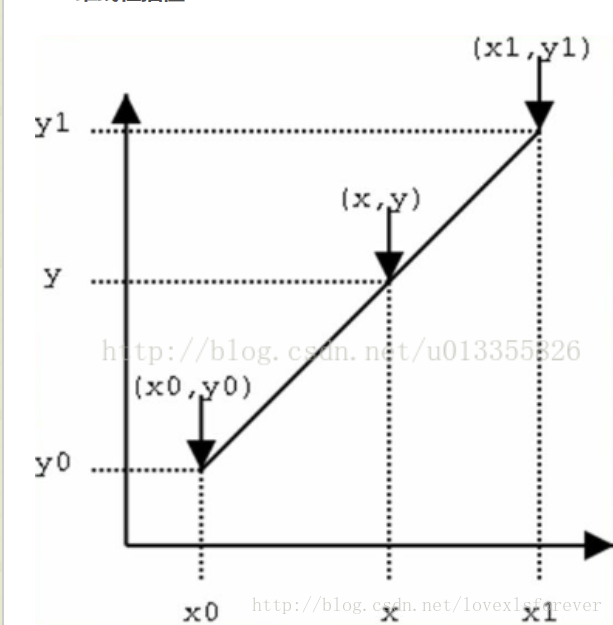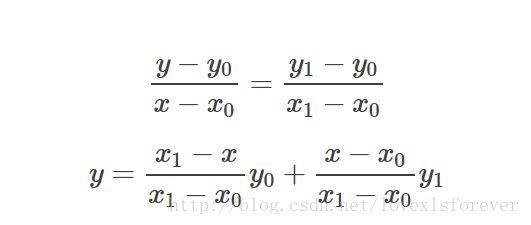上面比较好理解吧，仔细看就是用x和x0，x1的距离作为一个权重，用于y0和y1的加权。双线性插值本质上就是在两个方向上做线性插值。（其实就是有两个点确定的一次函数，然后在函数上去值呗）
双线性插值法
在数学上，双线性插值是有两个变量的插值函数的线性插值扩展，其核心思想是在两个方向分别进行一次线性插值。见下图：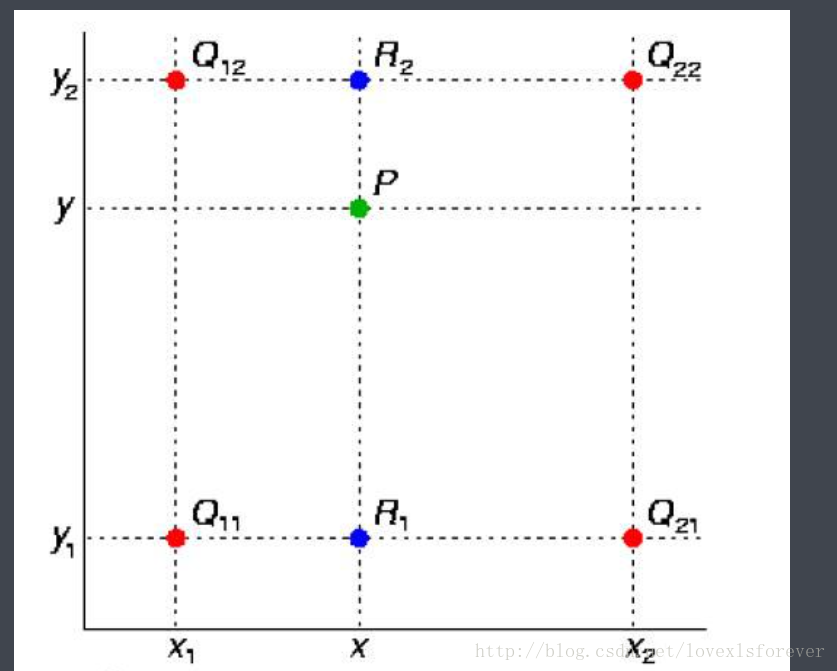假如我们想得到未知函数 f 在点 P = (x, y) 的值，假设我们已知函数 f 在 Q11 = (x1, y1)、Q12 = (x1, y2), Q21 = (x2, y1) 以及 Q22 = (x2, y2) 四个点的值。最常见的情况，f就是一个像素点的像素值。首先在 x 方向进行线性插值，得到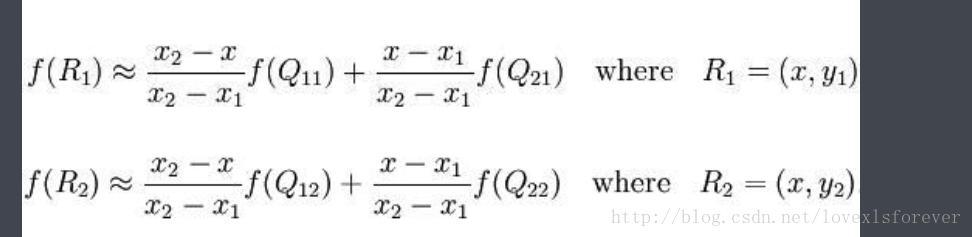然后在 y 方向进行线性插值，得到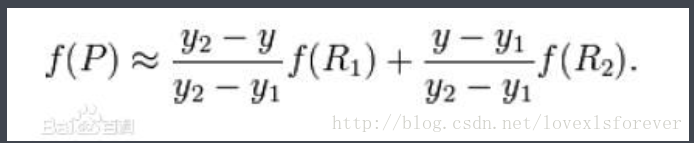综合起来就是双线性插值最后的结果：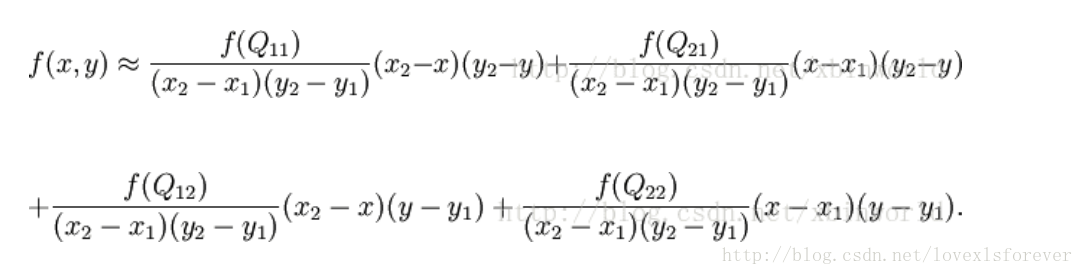由于图像双线性插值只会用相邻的4个点，因此上述公式的分母都是1。
使用双线性插值时要注意什么（存在的问题）？
这部分的前提是，你已经明白什么是双线性插值并且在给定源图像和目标图像尺寸的情况下，可以用笔计算出目标图像某个像素点的值。当然，最好的情况是你已经用某种语言实现了网上一大堆博客上原创或转载的双线性插值算法，然后发现计算出来的结果和matlab、openCV对应的resize（）函数得到的结果完全不一样。
那这个究竟是怎么回事呢？
其实答案很简单，就是坐标系的选择问题，或者说源图像和目标图像之间的对应问题。
按照网上一些博客上写的，源图像和目标图像的原点（0，0）均选择左上角，然后根据插值公式计算目标图像每点像素，假设你需要将一幅5x5的图像缩小成3x3，那么源图像和目标图像各个像素之间的对应关系如下：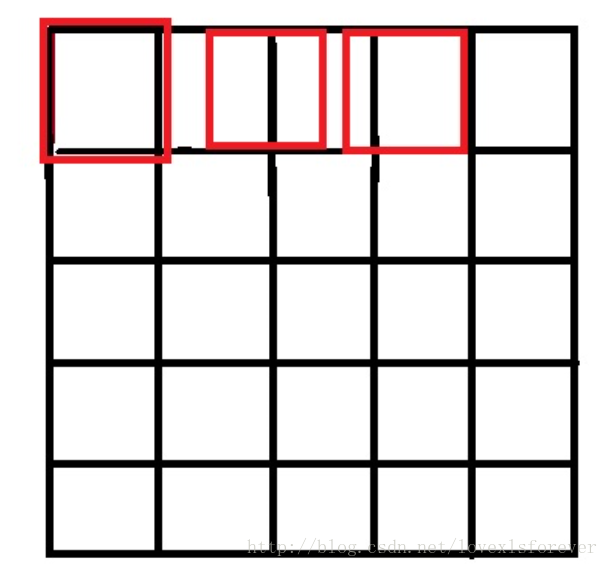只画了一行，用做示意，从图中可以很明显的看到，如果选择右上角为原点（0，0），那么最右边和最下边的像素实际上并没有参与计算，而且目标图像的每个像素点计算出的灰度值也相对于源图像偏左偏上。
那么，让坐标加1或者选择右下角为原点怎么样呢？很不幸，还是一样的效果，不过这次得到的图像将偏右偏下。
最好的方法就是，两个图像的几何中心重合（也就是下面要讲的优化策略），并且目标图像的每个像素之间都是等间隔的，并且都和两边有一定的边距，这也是matlab和openCV的做法。如下图：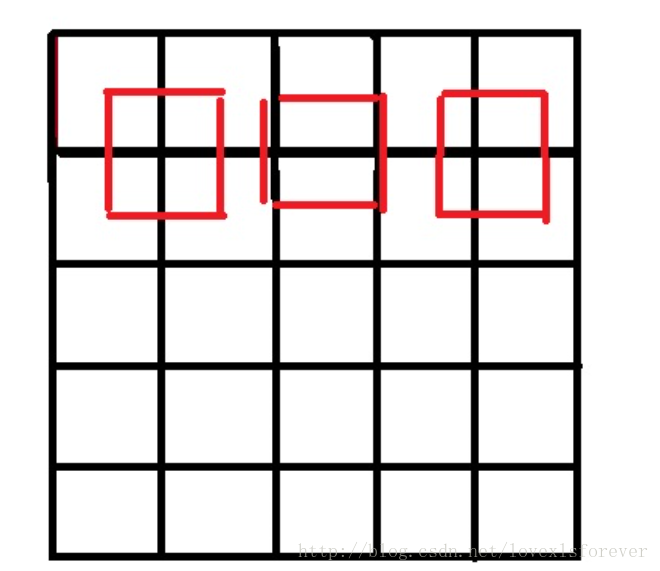如果你不懂我上面说的什么，没关系，只要在计算对应坐标的时候改为以下公式即可，
int x=(i+0.5)*m/a-0.5
int y=(j+0.5)*n/b-0.5
instead of
int x=im/a
int y=jn/b
利用上述公式，将得到正确的双线性插值结果
加速及优化策略
单纯按照上文实现的插值算法只能勉强完成插值的功能，速度和效果都不会理想，在具体代码实现的时候有些小技巧。参考OpenCV源码以及网上博客整理如下两点：
源图像和目标图像几何中心的对齐
将浮点运算转换成整数运算
源图像和目标图像几何中心的对齐
方法：在计算源图像的虚拟浮点坐标的时候，一般情况：
srcX=dstX* (srcWidth/dstWidth) ,
srcY = dstY * (srcHeight/dstHeight)
中心对齐：
SrcX=(dstX+0.5)* (srcWidth/dstWidth) -0.5
SrcY=(dstY+0.5) * (srcHeight/dstHeight)-0.5
原理：
双线性插值算法及需要注意事项这篇博客解释说“如果选择右上角为原点（0，0），那么最右边和最下边的像素实际上并没有参与计算，而且目标图像的每个像素点计算出的灰度值也相对于源图像偏左偏上。”我有点保持疑问。
将公式变形:
srcX=dstX *  (srcWidth/dstWidth)+0.5 * (srcWidth/dstWidth-1)
相当于我们在原始的浮点坐标上加上了0.5 * (srcWidth/dstWidth-1)这样一个控制因子，这项的符号可正可负，与srcWidth/dstWidth的比值也就是当前插值是扩大还是缩小图像有关，有什么作用呢？看一个例子：假设源图像是33，中心点坐标（1，1）目标图像是99，中心点坐标（4，4），我们在进行插值映射的时候，尽可能希望均匀的用到源图像的像素信息，最直观的就是（4,4）映射到（1,1）现在直接计算srcX=4 * 3/9=1.3333！=1，也就是我们在插值的时候所利用的像素集中在图像的右下方，而不是均匀分布整个图像。现在考虑中心点对齐，srcX=(4+0.5) * 3/9-0.5=1，刚好满足我们的要求。
将浮点运算转换成整数运算
参考图像处理界双线性插值算法的优化
直接进行计算的话，由于计算的srcX和srcY 都是浮点数，后续会进行大量的乘法，而图像数据量又大，速度不会理想，解决思路是：
浮点运算→→整数运算→→”<<左右移按位运算”。
放大的主要对象是u，v这些浮点数，OpenCV选择的放大倍数是2048“如何取这个合适的放大倍数呢，要从三个方面考虑，
第一：精度问题，如果这个数取得过小，那么经过计算后可能会导致结果出现较大的误差。
第二，这个数不能太大，太大会导致计算过程超过长整形所能表达的范围。
第三：速度考虑。假如放大倍数取为12，那么算式在最后的结果中应该需要除以12 * 12=144，但是如果取为16，则最后的除数为16 * 16=256，这个数字好，我们可以用右移来实现，而右移要比普通的整除快多了。”我们利用左移11位操作就可以达到放大目的。


展开全文• Python OpenCV 图像的双线性插值算法详细说明，最容易理解的博客python opencv 人工智能 机器学习
• 使用python对2维图像采用最邻近插值和双线性插值算法实现旋转
• 最近邻插值 与 双线性插值算法 优化迭代 的 0.5 像素之差python opencv 计算机视觉 人工智能 机器学习
• 双线性插值算法及实现 VC图象编程 双线性插值算法及实现 VC图象编程 双线性插值算法及实现 VC图象编程
• -3787- 0引言 数字图像处理的特点之一是处理的数据量大,对...算法复杂度是综合性能根本的制约因素,如何降低算法复杂度又不不会对数字图像处理的质量产生大的影响是一个研究热点[3-4] 最近邻点法双线性插值算法是广
• 针对单板CMOS图像传感器采集的Bayer模板的数据，结合FPGA硬件结构FIFO_DFF构成3×3插值模板，提出一种改进的双线性插值算法。插值过程中利用当前要还原的分量同通道的水平、垂直以及两个对角线方向上的已知分量进行...CMOS图像传感器
•图像处理 视觉slam
• ## 双线性插值算法的详细总结

万次阅读 多人点赞 2017-12-17 16:43:30
里面用到了图像的单应性矩阵变换，在最后的图像重映射，由于目标图像的坐标是非整数的，所以需要用到插值的方法，用的就是双线性插值，下面的博文主要是查看了前辈的博客对双线性插值算法原理进行了一个总结，在这里...
• 里面用到了图像的单应性矩阵变换，在最后的图像重映射，由于目标图像的坐标是非整数的，所以需要用到插值的方法，用的就是双线性插值，下面的博文主要是查看了前辈的博客对双线性插值算法原理进行了一个总结，在这里...
• 双线性插值算法原理 python实现 这个博主写的很好，指路--->https://www.cnblogs.com/wojianxin/p/12516029.html
• 图像插值目前，终端显示器都由点阵的像素点构成，在表现非水平或者竖直的直线时，必然会出现直线的歪曲，即锯齿效应。位图经过缩放、旋转等变换，都会使锯齿效应增强。尤其当显示分辨率过低时，在表现非水平或竖直的...
• 双线性插值算法及其实现，有源代码，感觉还不错，共享一下...